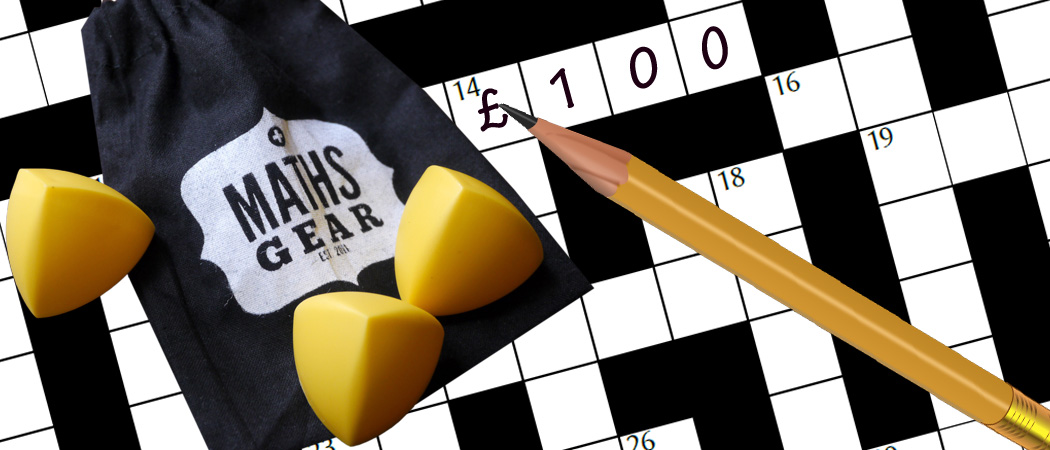# Prize crossnumber, Issue 11

£100 of MathsGear goodies to be won if you can solve itOur original prize crossnumber is featured on pages 56 and 57 of Issue 11.

### Rules

• Although many of the clues have multiple answers, there is only one solution to the completed crossnumber. As usual, no numbers begin with 0. Use of Python, OEIS, Wikipedia, etc. is advised for some of the clues.
• One randomly selected correct answer will win a £100 Maths Gear goody bag, including non-transitive dice, a Festival of the Spoken Nerd DVD, and much, much more. Three randomly selected runners up will win a Chalkdust T-shirt. Maths Gear is a website that sells nerdy things worldwide, with free UK shipping.
• To enter, enter the sum of the across clues below by 3 September 2020. Only one entry per person will be accepted. Winners will be notified by email and announced on our blog by 1 October 2020.

### Clues

#### Across

• 1 38A backwards. (10)
• 5 The product of the digits of this number is prime. (6)
• 8 A multiple of 10 that is equal to the difference between 29D and 12D. (2)
• 9 Equal to 1D. (2)
• 10 This number is equal to the sum of the cubes of its digits. (3)
• 13 The difference between 18A and 26A. (3)
• 15 This number is a multiple of each of its digits. (7)
• 17 20A multiplied by a factor of 17A. (3)
• 18 Each digit of this number (except the first) is one less than the previous digit. (3)
• 20 The sum of the digits of 6D. (2)
• 21 The final digit of this number is the same as the final digit of 10A and the first digit of this number is the same as the first digit of 24A. (2)
• 24 The final digit of this number is the same as the final digit of 33A and the first digit of this number is the same as the first digit of 21A. (2)
• 25 The sum of the digits of 19D. (2)
• 26 Each digit of this number (except the first) is one less than the previous digit. (3)
• 27 25A multiplied by a factor of 27A. (3)
• 29 This number is a multiple of each of its digits. (7)
• 32 The difference between 18A and 26A. (3)
• 33 This number is equal to the sum of the cubes of its digits. (3)
• 35 Equal to 37D. (2)
• 36 A multiple of 10 that is equal to the difference between 29D and 12D. (2)
• 37 The product of the digits of this number is prime. (6)
• 38 Larger than 1A, but divisible by 1A. (10)

#### Down

• 1 Equal to 9A. (2)
• 2 1D multiplied by 9A. (3)
• 3 One more than a multiple of 35D. (3)
• 4 Larger than 28D, but divisible by 28D. (6)
• 6 For each $n$ from 0 to 9, the digit $n$ appears either 0 or $n$ times in this number. (9)
• 7 This number is equal to the sum of the fifth powers of its digits. (5)
• 11 A non-square multiple of 6 that is equal to 3 more than the sum of the digits of 15A. (2)
• 12 A square number whose first digit is equal to the first digit of 15A. (2)
• 14 Larger than 16D, but divisible by 16D. (4)
• 16 14D backwards. (4)
• 19 For each $n$ from 0 to 9, the digit $n$ appears either 0 or $n$ times in this number. (9)
• 22 23D backwards. (4)
• 23 Larger than 22D, but divisible by 22D. (4)
• 28 4D backwards. (6)
• 29 A square number whose first digit is equal to the first digit of 29A. (2)
• 30 A non-square multiple of 6 that is equal to 3 more than the sum of the digits of 29A. (2)
• 31 This number is equal to the sum of the fifth powers of its digits. (5)
• 34 One more than a multiple of 2D. (3)
• 35 37D multiplied by 35A. (3)
• 37 Equal to 35A. (2)